Looking for VBA Tutorials?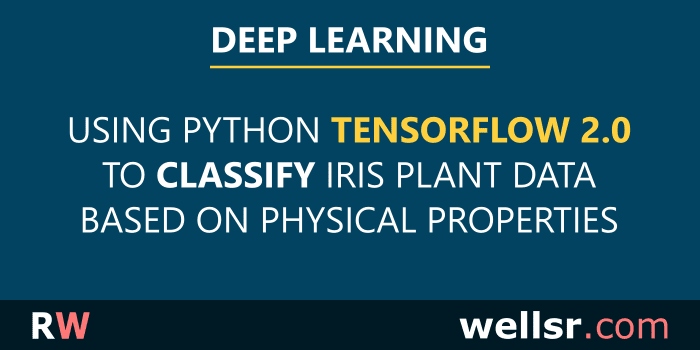#### Feb 23, 2020

This tutorial develops an Iris plant classification tool to explain how to perform classification tasks using Python's TensorFlow 2.0 library for deep learning.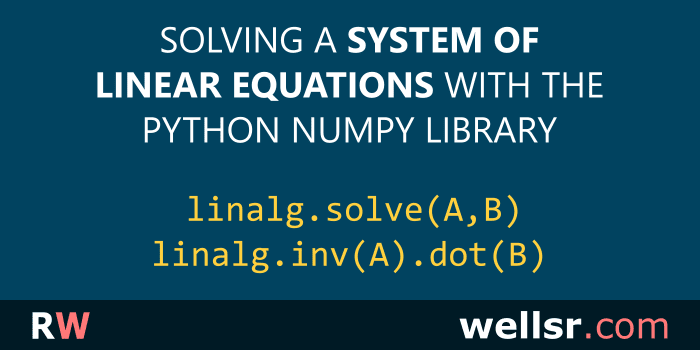#### Feb 7, 2020

This tutorial uses examples to explain how to solve a system of linear questions using Python's NumPy library and its linalg.solve and linalg.inv methods.#### \$120.00 \$57.00

Want to learn more programming languages? We've combined each of our comprehensive VBA reference guides into a single bundle with over 200 tips and macros covering the 125 most important topics in VBA.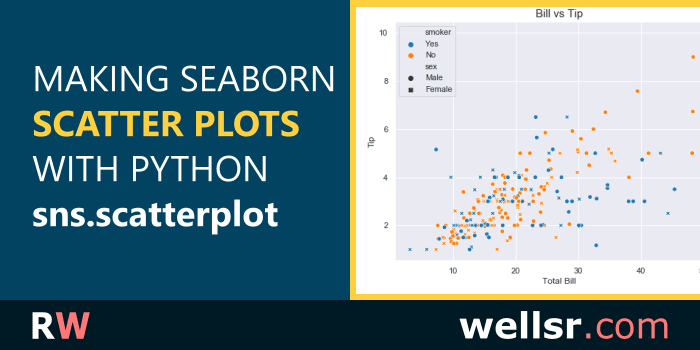#### Jan 17, 2020

The tutorial explains how to make different scatter plots using the Python Seaborn library. Several code examples demonstrate how to use sns.scatterplot.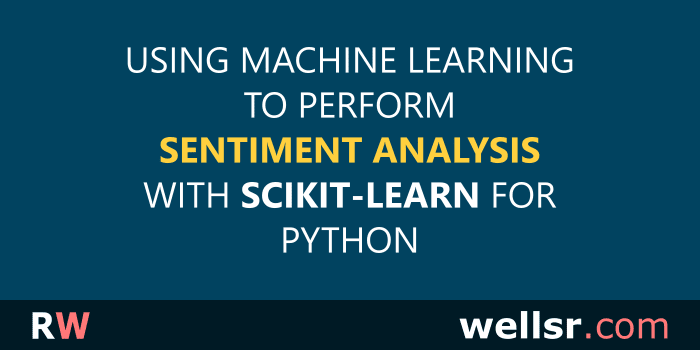#### Dec 20, 2019

This tutorial performs sentiment analysis using Python's Scikit-Learn library for machine learning. We use the sklearn library to analyze the sentiment of movie reviews.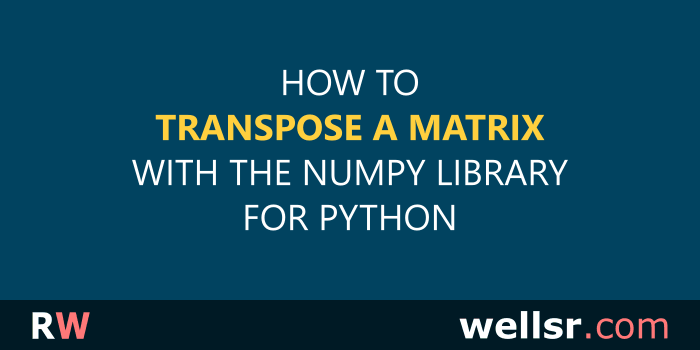#### Dec 13, 2019

This tutorial explains how to transpose a matrix using NumPy in Python and includes practical examples illustrating when you might need to transpose a matrix.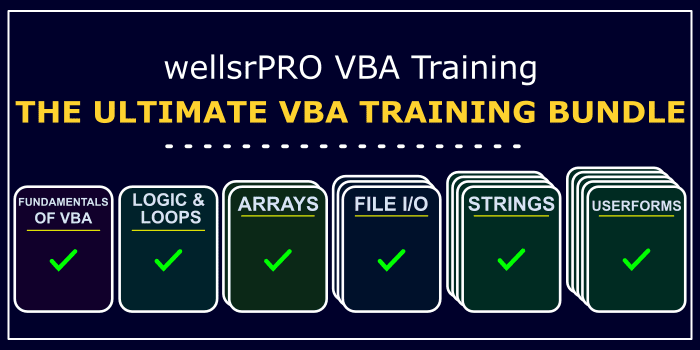#### \$120.00 \$57.00

We created a suite of 6 VBA cheat sheets with over 200 tips showing you everything you need to know to start making power Excel applications. Take a look!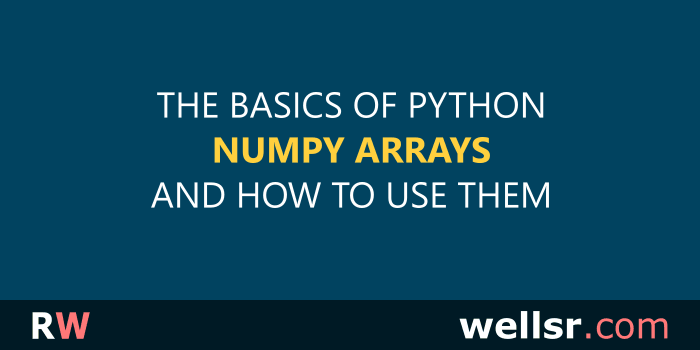#### Nov 27, 2019

This tutorial introduces the Python NumPy Library and explains how to use it to create arrays and perform arithmetic and matrix operations on NumPy arrays.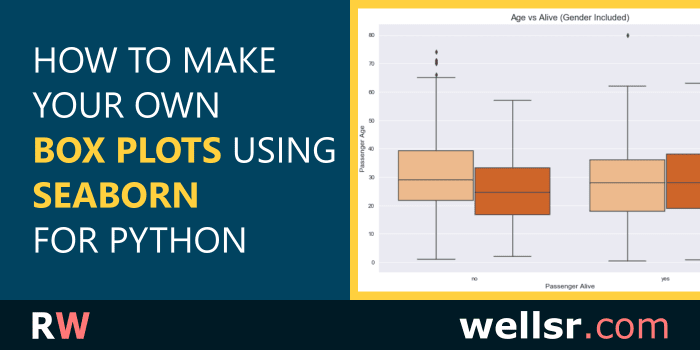#### Nov 22, 2019

This tutorial draws different Seaborn Boxplots using the Python Seaborn library. It includes examples for editing the colors, columns and labels of a box plot.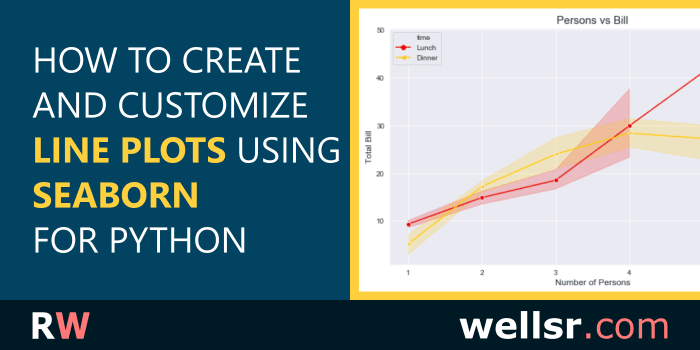#### Nov 12, 2019

This tutorial explains how to draw different line plots using the Python Seaborn library. It includes examples for setting line plot labels, markers and more.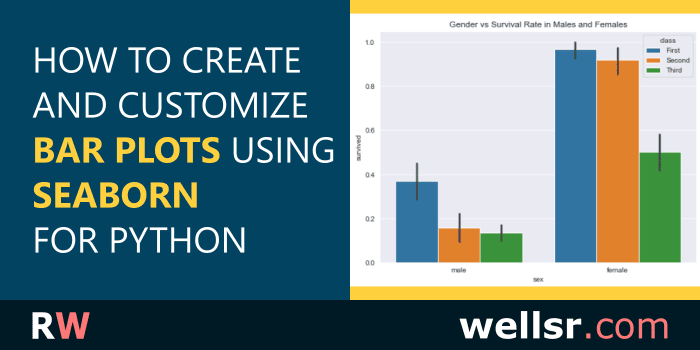#### Oct 25, 2019

This tutorial explains how to use the Seaborn barplot function in Python, including how to make grouped bar plots, bar plots with values and barplot titles.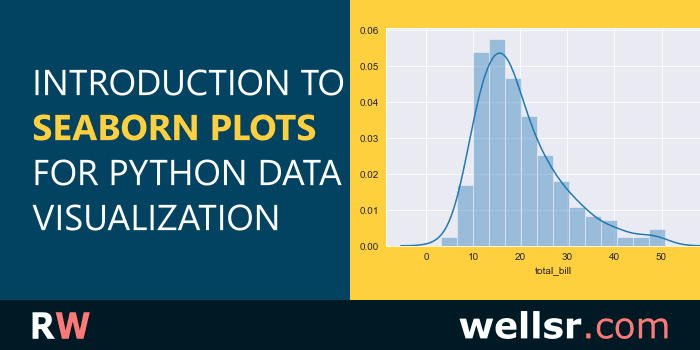#### Oct 18, 2019

This tutorial introduces the Python Seaborn library for data visualization and includes Seaborn plot examples so you can see how it helps visualize Python data.